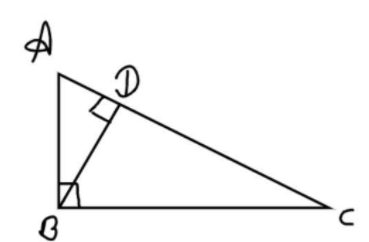Courses
Courses for Kids
Free study material
Free LIVE classes
MoreLIVE
Join Vedantu’s FREE Mastercalss

# Triangle has sides $5cm,{\text{ }}12cm,$ and $13cm$. Find the length of the perpendicular from the opposite vertex to the side whose length is $13cm$.Verified
363k+ views
Hint: In this question we will use the area of the right angle triangle that is half multiplied by its base and perpendicular, by considering the other base and perpendicular of the same triangle then we will equate both areas.Let $AB = 5cm,{\text{ }}BC = 12cm,{\text{ }}CA = 13cm$
So, these sides makes a right angle triangle because
${\left( {CA} \right)^2} = {\left( {AB} \right)^2} + {\left( {BC} \right)^2} \\ {13^2} = {5^2} + {12^2} = 169 = {13^2} \\$
Therefore ABC is a right angle triangle at B
Let BD be the perpendicular on side AC
Let $BD = xcm$
From figure the area of right angle triangle is half multiply by perpendicular time’s base
$\Delta ABC = \dfrac{1}{2}\left( {AB} \right)\left( {BC} \right) = \dfrac{1}{2}\left( {BD} \right)\left( {AC} \right) \\ = \dfrac{1}{2}\left( 5 \right)\left( {12} \right) = \dfrac{1}{2}\left( x \right)\left( {13} \right) \\ \Rightarrow 60 = 13x \\ \Rightarrow x = \dfrac{{60}}{{13}}cm \\$
So, this is the required perpendicular distance from the opposite vertex to the side whose length is $13cm$.

Note: In such types of questions first draw the pictorial representation of the given problem, then check whether it is right angle triangle or not if it is then using the formula of area of triangle which is half multiply by perpendicular time’s base, then we can easily calculated the length of the perpendicular from the opposite vertex to the side whose length is $13cm$.
Last updated date: 27th Sep 2023
Total views: 363k
Views today: 6.63k